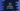# Java program to print the boundary elements of a matrix### Java program to print the boundary elements of a matrix :

In this tutorial, we will learn how to print the boundary elements of a matrix using Java. For example, for the below matrix :

``````1    2   3
4   5   6
7   8   9
``````

The matrix with boundary elements will be as below :

``````1    2   3
4       6
7   8   9
``````

### Algorithm to solve this problem :

1. First, ask the user to input the row and column number for the matrix.

2. Create one matrix with same row and column as the user entered.

3. Read all elements for the matrix.

4. Print the boundary elements of the user given matrix. Print the first row, last row, first column and last column elements. For other elements, print one blank.

### Java Program :

``````import java.util.Scanner;

class Main {
public static void main(String args[]) {
//1
int row, col;

//2
Scanner scanner = new Scanner(System.in);
System.out.println("Enter total number of rows : ");
row = scanner.nextInt();

//3
System.out.println("Enter total number of columns : ");
col = scanner.nextInt();

//4
int inputArray[][] = new int[row][col];

//5
for (int i = 0; i < row; i++) {
for (int j = 0; j < col; j++) {
System.out.println("Enter element for array[" + (i + 1) + "," + (j + 1) + "] : ");
inputArray[i][j] = scanner.nextInt();
}
}

//6
System.out.println("You have entered : ");
for (int i = 0; i < row; i++) {
for (int j = 0; j < col; j++) {
System.out.print(inputArray[i][j] + "\t");
}
System.out.println();
}

//7
System.out.println("The boundary elements of this matrix are : ");
for (int i = 0; i < row; i++) {
for (int j = 0; j < col; j++) {
if (i == 0 || j == 0 || i == row - 1 || j == col - 1) {
System.out.print(inputArray[i][j] + "\t");
} else {
System.out.print("\t");
}

}
System.out.println();
}
}
}
``````

### Explanation :

The commented numbers in the above program denote the step-number below :

1. Create two integer variables row and col to store the row and column count for the matrix.

2. Create one Scanner object to read user input numbers. Ask the user to enter the total number of rows for the matrix and save it in row variable.

3. Similarly, ask the user to enter the total number of columns for the matrix and save it in col variable.

4. Create one two dimensional integer array of size [row][column] using the user input values.

5. Run two for loops to read all elements for the matrix. Read and store it in the two dimensional array.

6. After all items are stored, print out all elements again using two for loops.

7. Now, print out the boundary elements of the matrix. We have two for loops here. The outer loop runs from i=0 to i=row - 1 and the inner loop runs from j=0 to j=col-1. We need to print the elements for first row,last row, first column and last column. Print these elements and for other elements, print one tab (‘\t’).

### Sample Output :

``````Enter total number of rows :
3
Enter total number of columns :
3
Enter element for array[1,1] :
1
Enter element for array[1,2] :
2
Enter element for array[1,3] :
3
Enter element for array[2,1] :
4
Enter element for array[2,2] :
5
Enter element for array[2,3] :
6
Enter element for array[3,1] :
7
Enter element for array[3,2] :
8
Enter element for array[3,3] :
9
You have entered :
1   2   3
4   5   6
7   8   9
The boundary elements of this matrix are :
1   2   3
4       6
7   8   9
``````